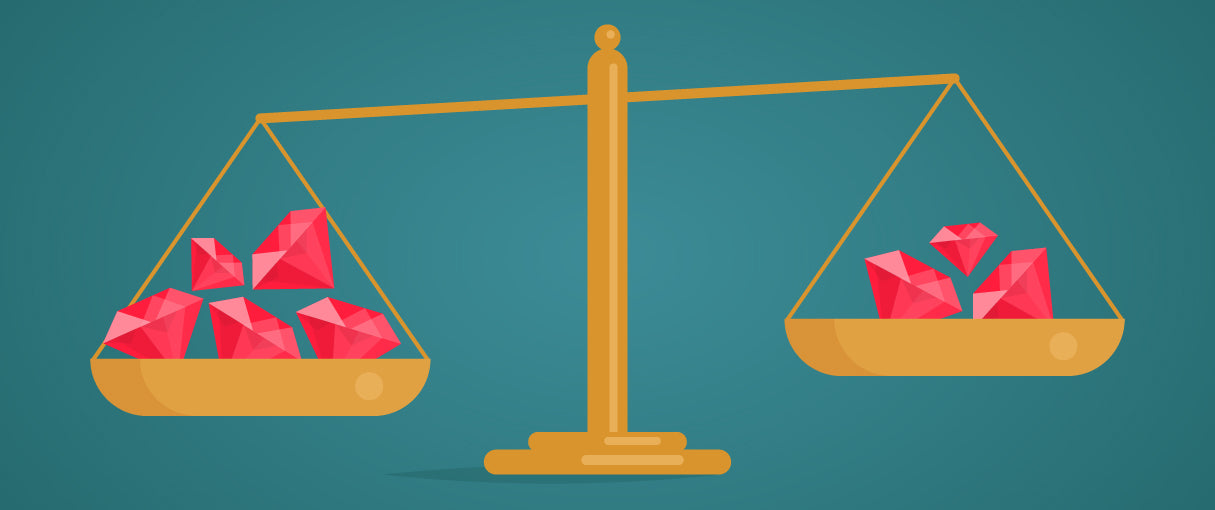Jiazhen Xie's blog

# Ruby equality

By Jiazhen Xie on Nov 3, 2013The Object class defines three methods related to equality ? `==`, `eql?` and `equals?`.

#### ==, eql?

Both == and eql? implement value equality checks ? they are not interested in whether two variables point to the same object in memory, but whether two objects are equal in terms of their values.

#### equal?

It implements what?s commonly known as reference equality check. The method returns true only if its receiver (the object upon the method was invoked) and parameter (the object we?re comparing to) are the same object (Java developers should think of the `==` operator there).

``````some_word = "word"
some_other_word = some_word

some_word.equal? some_other_word # true``````